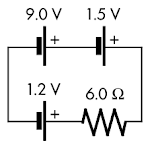## 20140405

### Physics quiz question: "backwards" emf

Physics 205B Quiz 4, spring semester 2014
Cuesta College, San Luis Obispo, CA

Cf. Giambattista/Richardson/Richardson, Physics, 2/e, Problem 18.37Three ideal emf sources are connected to a resistor, as shown at right. The current flowing through the resistor is:
(A) 1.6 A.
(B) 1.8 A.
(C) 2.0 A.
(D) 9.3 A.

Correct answer (highlight to unhide): (A)

The equivalent emf of this circuit is the sum of these emfs, however the polarity of the 1.2 V emf source is "backwards" with respect to the 9.0 V and the 1.5 V emf sources. Thus the equivalent emf is given by:

Veq = –1.2 V + 9.0 V + 1.5 V = 9.3 V.

Thus the current flowing through all parts of this series circuit is given by:

I = ∆Veq/R = (9.3 V)/(6.0 Ω) = 1.55 A,

which to two significant figures is 1.6 A.

(Response (B) is (+9.0 V + 1.5 V)/(6.0 Ω); response (C) is (1.2 V +9.0 V + 1.5 V)/(6.0 Ω); response (D) is (–1.2 V +9.0 V + 1.5 V).)

Sections 30882, 30883
Exam code: quiz04mCnC
(A) : 11 students
(B) : 4 students
(C) : 18 students
(D) : 3 students

Success level: 31%
Discrimination index (Aubrecht & Aubrecht, 1983): 0.28